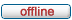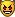All times are UTC - 5 hours [ DST ]

Author Message
 Post subject: Re: Which NAND flash reader ?Posted: May 31st, 2020, 14:24Joined: October 24th, 2009, 15:22
Posts: 685
Location: Poland
Not bad. 75% map is green_________________
Odzyskiwanie danych

TopPost subject: Re: Which NAND flash reader ?Posted: May 31st, 2020, 15:22Joined: May 12th, 2020, 11:27
Posts: 39
Location: France
Ok !

I try to read this block multiple time to see if some bits are changing

TopPost subject: Re: Which NAND flash reader ?Posted: June 1st, 2020, 2:37Joined: September 23rd, 2019, 2:55
Posts: 6
Location: Poland
nlc wrote:
I have some bad bits in the dump :
But I think the dump quality is good, because the white column is 12 bits wide, and on a dump sample of 8192 pages, thus 98304 bits, I counted only 89 bad bits (0.00091%)
If I'm not mistaken, the 70 bytes ECC for 1024 data byte (total 8752 bits) can correct up to 40 bit errors, thus up to 40/8752 = 0.004%

But what if, let's say you have 1 bit error every 12 bits in page, then in theory you can have (1094*8)/12= 682 error bits per chunk (data+ecc).
ps. you not mistaken about how many bits you can correct, is 40.

TopPost subject: Re: Which NAND flash reader ?Posted: June 1st, 2020, 3:20Joined: May 12th, 2020, 11:27
Posts: 39
Location: France
Gregory wrote:

But what if, let's say you have 1 bit error every 12 bits in page, then in theory you can have (1094*8)/12= 682 error bits per chunk (data+ecc).

Of course, but in this case statistically I should see more page with bad pixel in the 12 bits blank I think

Today I need to work on ecc to be able to check by myself the pages dump quality

TopPost subject: Re: Which NAND flash reader ?Posted: June 1st, 2020, 11:46Joined: May 12th, 2020, 11:27
Posts: 39
Location: France
ECC CALCULATION MAKES ME CRAZY !!!TopPost subject: Re: Which NAND flash reader ?Posted: June 2nd, 2020, 17:40Joined: May 12th, 2020, 11:27
Posts: 39
Location: France
This fuc***g chip is really complex !!
Will try to understand ECC later, today I tried to find how data are arranged in the nand, because from above screen capture it's clear the spare area is completely scrambled, pages are interleaved or something like that.

We know that generally all the Spare Area of a block have same values.
What I have done is to randomly take a 32 bytes spare area, and wrote a small utility to scan all the pages of the nand, and display all pages number which have at least 245 bits in common (to remove maching problem due to flipped bit). It worked very well, was able to understand how the data are arranged :

Code:
0032.001 = 256 common bits
0048.001 = 255 common bits
0064.001 = 256 common bits
0096.001 = 256 common bits
0112.001 = 256 common bits
0128.001 = 255 common bits
0144.001 = 255 common bits
0160.001 = 256 common bits
0192.001 = 256 common bits
0208.001 = 256 common bits
0224.001 = 255 common bits
0240.001 = 256 common bits
0256.001 = 256 common bits
0272.001 = 255 common bits
0288.001 = 255 common bits
0304.001 = 256 common bits
0320.001 = 256 common bits
0336.001 = 256 common bits
0352.001 = 255 common bits
0368.001 = 255 common bits
0384.001 = 256 common bits
0400.001 = 253 common bits
0416.001 = 256 common bits
0432.001 = 256 common bits
0448.001 = 255 common bits
0464.001 = 256 common bits
0480.001 = 255 common bits
0496.001 = 254 common bits
0512.001 = 255 common bits
0576.001 = 256 common bits
0592.001 = 255 common bits
0608.001 = 255 common bits
0624.001 = 255 common bits
0656.001 = 256 common bits
0704.001 = 256 common bits
0720.001 = 256 common bits
0736.001 = 254 common bits
0752.001 = 256 common bits
0768.001 = 255 common bits
0784.001 = 254 common bits
0816.001 = 256 common bits
0832.001 = 252 common bits
0848.001 = 256 common bits
0864.001 = 255 common bits
0880.001 = 255 common bits
0896.001 = 256 common bits
0912.001 = 254 common bits
0928.001 = 256 common bits
0960.001 = 254 common bits
0976.001 = 255 common bits
0992.001 = 255 common bits
1008.001 = 256 common bits
1024.001 = 255 common bits
1040.001 = 255 common bits
1056.001 = 256 common bits
1072.001 = 254 common bits
1088.001 = 255 common bits
1104.001 = 256 common bits
1120.001 = 256 common bits
1136.001 = 256 common bits
1152.001 = 255 common bits
1168.001 = 255 common bits
1184.001 = 254 common bits
1200.001 = 254 common bits
1216.001 = 256 common bits
1232.001 = 255 common bits
1248.001 = 256 common bits
1264.001 = 255 common bits
1280.001 = 255 common bits
1296.001 = 256 common bits
1328.001 = 256 common bits
1344.001 = 256 common bits
1360.001 = 256 common bits
1376.001 = 255 common bits
1392.001 = 256 common bits
1424.001 = 255 common bits
1440.001 = 256 common bits
1456.001 = 256 common bits
1472.001 = 256 common bits
1488.001 = 255 common bits
1504.001 = 256 common bits
1520.001 = 256 common bits
1536.001 = 256 common bits
1552.001 = 256 common bits
1568.001 = 255 common bits
1584.001 = 256 common bits
1600.001 = 256 common bits
1616.001 = 255 common bits
1632.001 = 256 common bits
1648.001 = 255 common bits
1664.001 = 256 common bits
1680.001 = 254 common bits
1696.001 = 256 common bits
1728.001 = 255 common bits
1744.001 = 254 common bits
1760.001 = 256 common bits
1776.001 = 255 common bits
1792.001 = 254 common bits
1808.001 = 255 common bits
1824.001 = 255 common bits
1840.001 = 255 common bits
1888.001 = 256 common bits
1904.001 = 255 common bits
1920.001 = 254 common bits
1936.001 = 256 common bits
1952.001 = 253 common bits
1968.001 = 256 common bits
1984.001 = 256 common bits
2000.001 = 256 common bits
2016.001 = 255 common bits
2048.001 = 256 common bits
2064.001 = 255 common bits
2080.001 = 256 common bits
535552 pages read analysed, 113 Spare Area are matching the pattern model !

XXXX.YYY is block_number.page_number

We can see all page containing the same spare area (and probably must be assembled together), are on the same page number, but every 16 block !

And my SA test pattern doesn't match at all on the dump of the second chip, it means data are not interleaved between nand chip I think.

Ok, now I write a small utility program to rearrange data, and see the result in GimpTopPost subject: Re: Which NAND flash reader ?Posted: June 2nd, 2020, 18:32Joined: May 13th, 2019, 7:50
Posts: 133
Location: Nederland
Ever come across this, very similar adventure: https://joshuawise.com/projects/ndfreco ... extraction

_________________
www.disktuna.com - photo repair service

TopPost subject: Re: Which NAND flash reader ?Posted: June 2nd, 2020, 19:35Joined: May 12th, 2020, 11:27
Posts: 39
Location: France
Yes thank you, already found this excellent articleTopPost subject: Re: Which NAND flash reader ?Posted: June 4th, 2020, 2:20Joined: September 23rd, 2019, 2:55
Posts: 6
Location: Poland
nlc wrote:
Attachment:
Screenshot_20200531-011656__01__01.jpg

I tested this emmc chip via NAND protocol on VNR and FE with and without VCC, VCCQ, VDDI capacitors, no visible differences in reading quality. You have big luck, you chip is in very good contition.

TopPost subject: Re: Which NAND flash reader ?Posted: June 6th, 2020, 3:45Joined: May 12th, 2020, 11:27
Posts: 39
Location: France
Received yesterday a working H26M42003GMRA. I filled it with 0x00 and can extract the Xor key now.

Will also be more easy to understand ECC

TopPost subject: Re: Which NAND flash reader ?Posted: June 6th, 2020, 20:36Joined: May 12th, 2020, 11:27
Posts: 39
Location: France
First file successfully recoveredTopPost subject: Re: Which NAND flash reader ?Posted: June 6th, 2020, 20:53Joined: December 4th, 2012, 1:35
Posts: 3540
nice work! Was it from the other partition you couldn't access before?

TopPost subject: Re: Which NAND flash reader ?Posted: June 7th, 2020, 3:30Joined: May 12th, 2020, 11:27
Posts: 39
Location: France
Yes, the first file I recovered was in the logical partition which was all 0x00.
I also found other files, but they contain errors, I need to implement ECC, I spent hours an hours and I haven't found how the ECC is calculated for now

TopPost subject: Re: Which NAND flash reader ?Posted: June 7th, 2020, 10:21Joined: May 12th, 2020, 11:27
Posts: 39
Location: France
FINISHED ! I successfully recovered all files I was looking forI spent a lot of hours and nights was short but it was a great experience, I learned a lot of thingsTopPost subject: Re: Which NAND flash reader ?Posted: June 7th, 2020, 11:22Joined: December 4th, 2012, 1:35
Posts: 3540
Great to hear. Very inspiring watching this unfold. Goes to show what hard work and learning can achieve.

TopPost subject: Re: Which NAND flash reader ?Posted: June 8th, 2020, 10:29Joined: May 13th, 2019, 7:50
Posts: 133
Location: Nederland
Ow wow, that's awesome!

_________________
www.disktuna.com - photo repair service

TopPost subject: Re: Which NAND flash reader ?Posted: July 3rd, 2020, 18:30Joined: May 12th, 2020, 11:27
Posts: 39
Location: France
The eMMC of my car died tooAnd the luck is not on my side this time, because the data quality on the 2 die are really really bad.
From the 2 dies dump I found 2 occurrences of one file I am looking for, and 5 occurrences of the second file I am looking for.

But data are so damaged that I am unable to reconstruct the 2 filesSo I looked on Read Retry and implemented it in my home made nand reader, based on a customized nand_hynix.c from Linux kernel as base code.
I know my read retry implementation works well because :
- I can get the Read Retry OTP data. From the eight time repeated 64 byte + 64 inverted byte Read Retry data, I can successfully get the eight data byte configuration for the eight Retry Mode.
- I found a page filled with 0xFF with some bit flip errors (26 bits error) when reading without Read Retry, and by changing read retry mode I can lower the bit errors to 8 (%70 less errors)

BUT !

On the pages where my important files data are, changing read retry mode doesn't improve anything !!Any idea why ? For people who do data recovery, how this behavior can happen?
Should I try to use another values in the eight read retry registers ?

I also found some filled 0xFF pages with a lot of bit flip, and also no RR mode from the eight available improved anything :/

TopDisplay posts from previous: All posts1 day7 days2 weeks1 month3 months6 months1 year Sort by AuthorPost timeSubject AscendingDescending

 All times are UTC - 5 hours [ DST ]

#### Who is online

Users browsing this forum: No registered users and 12 guests

 You cannot post new topics in this forumYou cannot reply to topics in this forumYou cannot edit your posts in this forumYou cannot delete your posts in this forumYou cannot post attachments in this forum

Search for:
 Jump to:  Select a forum ------------------ Knowledge base Conventional hard drives Flash storage, SSD Forensics Research and development Software and hardware tools Atola Technology support forum PC-3000 support forum SalvationData support forum DFL support forum HddSurgery support forum Rusolut support forum MRT Lab support forum Commercial manuals and solutions Employment offers and requests Market Market - large lots Lounge Fun stuff